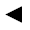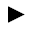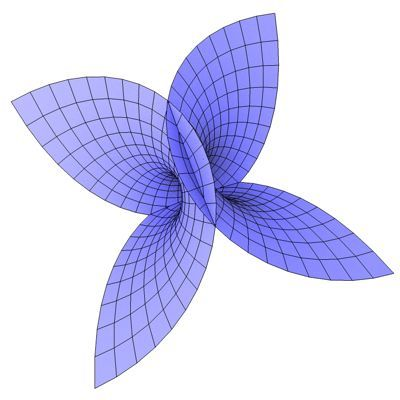п»їп»їп»ї math handbook calculator - Fractional Calculus Computer Algebra System software+++=п»ї[back]

## 3.6. Enneper's Surface

[next]The Enneper Surface [ 3,7,11 ] is represented by the following equations.

 x = u - (u 3 /3) + uv 2 ) 3-24 y = v - (v 3 /3) + u 2 v) 3-25 z = u2 - v2 3-26

To represent the area, the two parameters u and v can assume the following values ​​(domain of definition), for example.

 u is an element from the set of numbers [-2, 2] v is an element from the number set [-2, 2]

The Enneper's Surface is an open figure, so the definition range can be changed with the plugin.Fig. 10